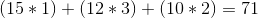Calculus 3 : Dot Product

Example Questions

Example Question #141 : Dot Product

Find the dot product between the vectors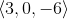and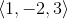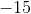Explanation:

To find the dot product between vectors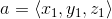and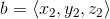, you apply the following formula: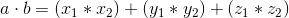. Applying to the vectors from the problem statement, we get: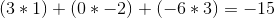Example Question #142 : Dot Product

Find the dot product between the vectors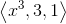and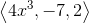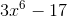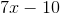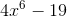Explanation:

To find the dot product between vectorsand, you apply the following formula:. Applying to the vectors from the problem statement, we get: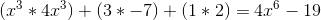Example Question #143 : Dot Product

Find the dot product of the two vectors: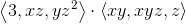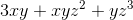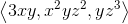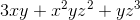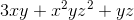Explanation:

The dot product of two vectors is given by the sum of the products of the corresponding components (for example,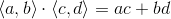)Example Question #144 : Dot Product

What is the product of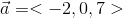and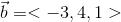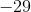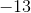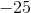Explanation: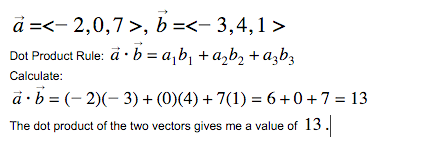Example Question #145 : Dot Product

Evaluate the dot product of the vectors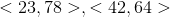.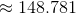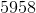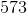Explanation:

To evaluate the dot product of any two vectors, we multiply them component-wise.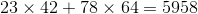.

Example Question #146 : Dot Product

Solve: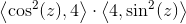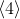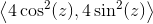Explanation:

The dot product of two vectors is given by the sum of the products of their respective components (for example,)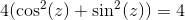Example Question #147 : Dot Product

Evaluate the dot product of the two vectors.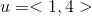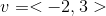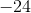Explanation:

The dot product for the vectors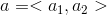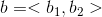is defined as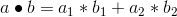For the vectors in this problem we find that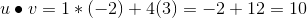Example Question #148 : Dot Product

Evaluate the dot product of the two vectors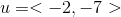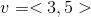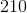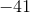Explanation:

The dot product for the vectorsis defined asFor the vectors in this problem we find that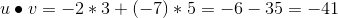Example Question #149 : Dot Product

Find the dot product between the vectors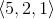and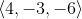Explanation:

To find the dot product between two vectorsand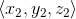, we use the formulaUsing the vectors from the problem statement, we get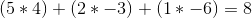Example Question #150 : Dot Product

Find the dot product between the vectors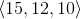and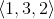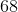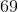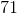Explanation:

To find the dot product between two vectorsand, we use the formulaUsing the vectors from the problem statement, we get